# New to Qlik Sense

If you’re new to Qlik Sense, start with this Discussion Board and get up-to-speed quickly.

Announcements
QlikWorld 2023, a live, in-person thrill ride. Save \$300 before February 6: REGISTER NOW!
cancel
Showing results for
Did you mean: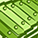Contributor III

## Edit Expression Error with Variable and if statement

I have been using a variable to scale my numbers to Billions, Millions and Thousands etc.

This works fine except when I introduce an if statement.

For instance this works great (Number formatting - Measure Expression):

\$(vScaleNumber(sum([Req Estimated Total Value]))) --> \$2.8B or \$1.7M etc...

I usually just take my number and wrap it in a variable \$(vScaleNumber(sum(XXX))) but when I introduce an If statement I get an error in the expression editor.

=\$(vScaleNumber(sum(if(isnull([Calc - Approval Days]), 0, [Req Estimated Total Value]))))

Error in expression:')' or';' expected

Any ideas would be appreciated.

Labels (1)
• ### Qlik Sense Expression Syntax

1 Solution

Accepted Solutions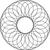Creator II

To make it work I had to load the variables from an external file.

As an example I used an xlsx file but you could have a table in some database with all your variables.

``````SET NullInterpret = 'NULL';

Test:
NOCONCATENATE
INLINE [
ReqID|"Calc - Req Post RAD Approval Days"|"Req Estimated Total Value"
RQMT-00017|3|100000034
RQMT-00015|NULL|3456789
RQMT-00011|56|100000
RQMT-00010|12|1000000
RQMT-00009|NULL|567000
RQMT-00005|2|1000
] (delimiter is '|');

Variables:
NOCONCATENATE
variable_name,
Replace(variable_exp, '\$(', '?(') AS variable_exp
(ooxml, embedded labels, table is Variables);

LET i_to = NoOfRows('Variables') - 1;

FOR i = 0 TO i_to

LET varname = Peek('variable_name', \$(i), 'Variables');
LET varexpr = Peek('variable_exp',  \$(i), 'Variables');

LET \$(varname) = Replace('\$(varexpr)', Chr(63) & Chr(40), Chr(36) & Chr(40));

NEXT

LET i_to 	=;
LET i 	 	=;
LET varname =;
LET varexpr =;

DROP TABLE Variables;``````
8 RepliesSpecialist II

How is vScaleNumber defined?Creator II

The issue is generated by the commas of the expression you are passing as argument to your variable.

The vScaleNumber variable expects a single argument (\$1) but "understands" that you are passing three to it.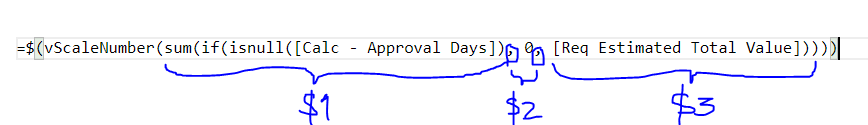You could solve the problem applying the technique explained in the following link:

https://community.qlik.com/t5/QlikView-Documents/Comma-problem-workaround-for-dollar-sign-expansion-...Contributor III
Author

JGMDataAnalysis thanks for the insight. I now know what the issue is but how is the syntax structured?

// Definition for Scaling numbers
SET vScaleNumber=if(\$1>=1000000000, dual(num(\$1/1000000000,'\$#,##0.0B'),\$1),if(\$1>=1000000, dual(num(\$1/1000000,'\$#,##0.0M'),\$1),if(\$1>=1000, dual(num(\$1/1000,'\$#,##0.0K'),\$1),
if(\$1<=-1000000000, dual(num(\$1/1000000000,'\$#,##0.0B'),\$1),if(\$1<=-1000000, dual(num(\$1/1000000,'\$#,##0.0M'),\$1),if(\$1<=-1000, dual(num(\$1/1000,'\$#,##0.0K'),\$1),num(\$1,'\$#,##0.0')))))));

Usage as KPI measure: Error

\$(vScaleNumber(sum(if(IsNull([Calc - Req Post RAD Approval Days]), 0, [Req Estimated Total Value]))))

Using Replace workaround SUM({\$< \$(=REPLACE('\$1', ';', ',')) >} quantity) : ????Specialist II

Can you do it the other way round?

=if(isnull([Calc - Approval Days]), vScaleNumber(0),vScaleNumber(sum( [Req Estimated Total Value]))Creator II

For your particular case you could use the solution proposed by @agolorenzoconforti or apply the following ...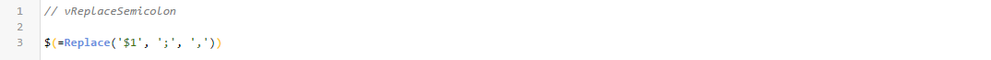Variable Call:

\$(vScaleNumber(Sum(If(IsNull([Calc - Req Post RAD Approval Days]); 0; [Req Estimated Total Value]))))

In the variable call take the precaution of replacing the commas with semicolons!!!Contributor III
Author

@JGMDataAnalysis, I still can't get this to work.

Create 2 variables:

SET vReplaceSemicolon=\$(=Replace('\$1', ';', ','));

SET vScaleNumberNew=If(fAbs(\$(vReplaceSemicolon(\$1))) >= Pow(10,9), Dual(Num(\$(vReplaceSemicolon(\$1)) / Pow(10, 9), '\$#,##0.0B'), \$(vReplaceSemicolon(\$1))),
If(fAbs(\$(vReplaceSemicolon(\$1))) >= Pow(10,6), Dual(Num(\$(vReplaceSemicolon(\$1)) / Pow(10, 6), '\$#,##0.0M'), \$(vReplaceSemicolon(\$1))),
If(fAbs(\$(vReplaceSemicolon(\$1))) >= Pow(10,3), Dual(Num(\$(vReplaceSemicolon(\$1)) / Pow(10, 3), '\$#,##0.0K'), \$(vReplaceSemicolon(\$1))),
Dual(Num(\$(vReplaceSemicolon(\$1)), '\$#,##0.0'), \$(vReplaceSemicolon(\$1))))));

// When you inspect the vScaleNumberNew there is still an error//Create Test Data:

ReqID|"Calc - Req Post RAD Approval Days"|"Req Estimated Total Value"
RQMT-00017|3|100000034
RQMT-00015| |3456789
RQMT-00011|56|100000
RQMT-00010|12|1000000
RQMT-00009| |567000
RQMT-00005|2|1000
] (delimiter is '|');

//Create KPIs:

\$(vScaleNumberNew(Sum(If(IsNull([Calc - Req Post RAD Approval Days]); 0; [Req Estimated Total Value]))))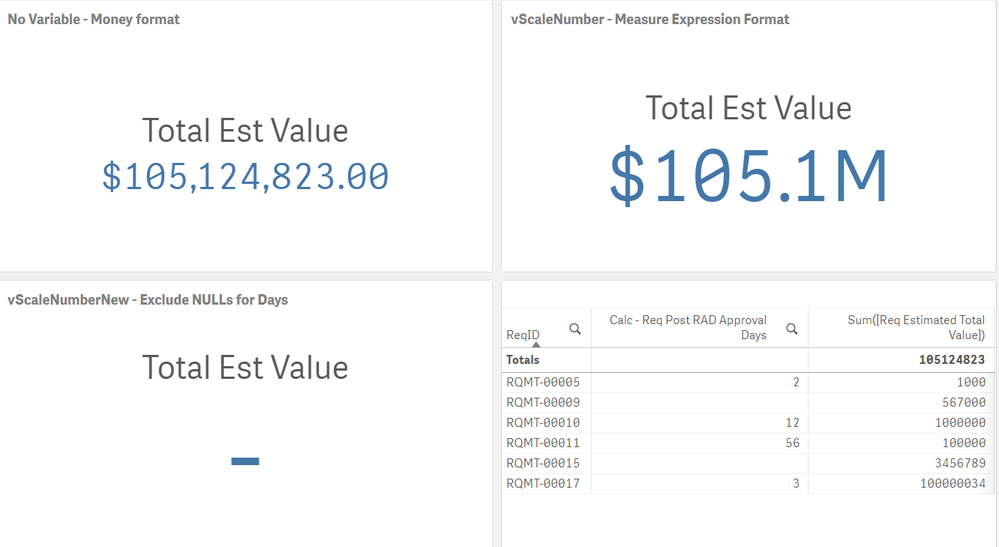Creator II

To make it work I had to load the variables from an external file.

As an example I used an xlsx file but you could have a table in some database with all your variables.

``````SET NullInterpret = 'NULL';

Test:
NOCONCATENATE
INLINE [
ReqID|"Calc - Req Post RAD Approval Days"|"Req Estimated Total Value"
RQMT-00017|3|100000034
RQMT-00015|NULL|3456789
RQMT-00011|56|100000
RQMT-00010|12|1000000
RQMT-00009|NULL|567000
RQMT-00005|2|1000
] (delimiter is '|');

Variables:
NOCONCATENATE
variable_name,
Replace(variable_exp, '\$(', '?(') AS variable_exp
(ooxml, embedded labels, table is Variables);

LET i_to = NoOfRows('Variables') - 1;

FOR i = 0 TO i_to

LET varname = Peek('variable_name', \$(i), 'Variables');
LET varexpr = Peek('variable_exp',  \$(i), 'Variables');

LET \$(varname) = Replace('\$(varexpr)', Chr(63) & Chr(40), Chr(36) & Chr(40));

NEXT

LET i_to 	=;
LET i 	 	=;
LET varname =;
LET varexpr =;

DROP TABLE Variables;``````Contributor III
Author

Thank you for your assistance JGM.Tags
Community Browser